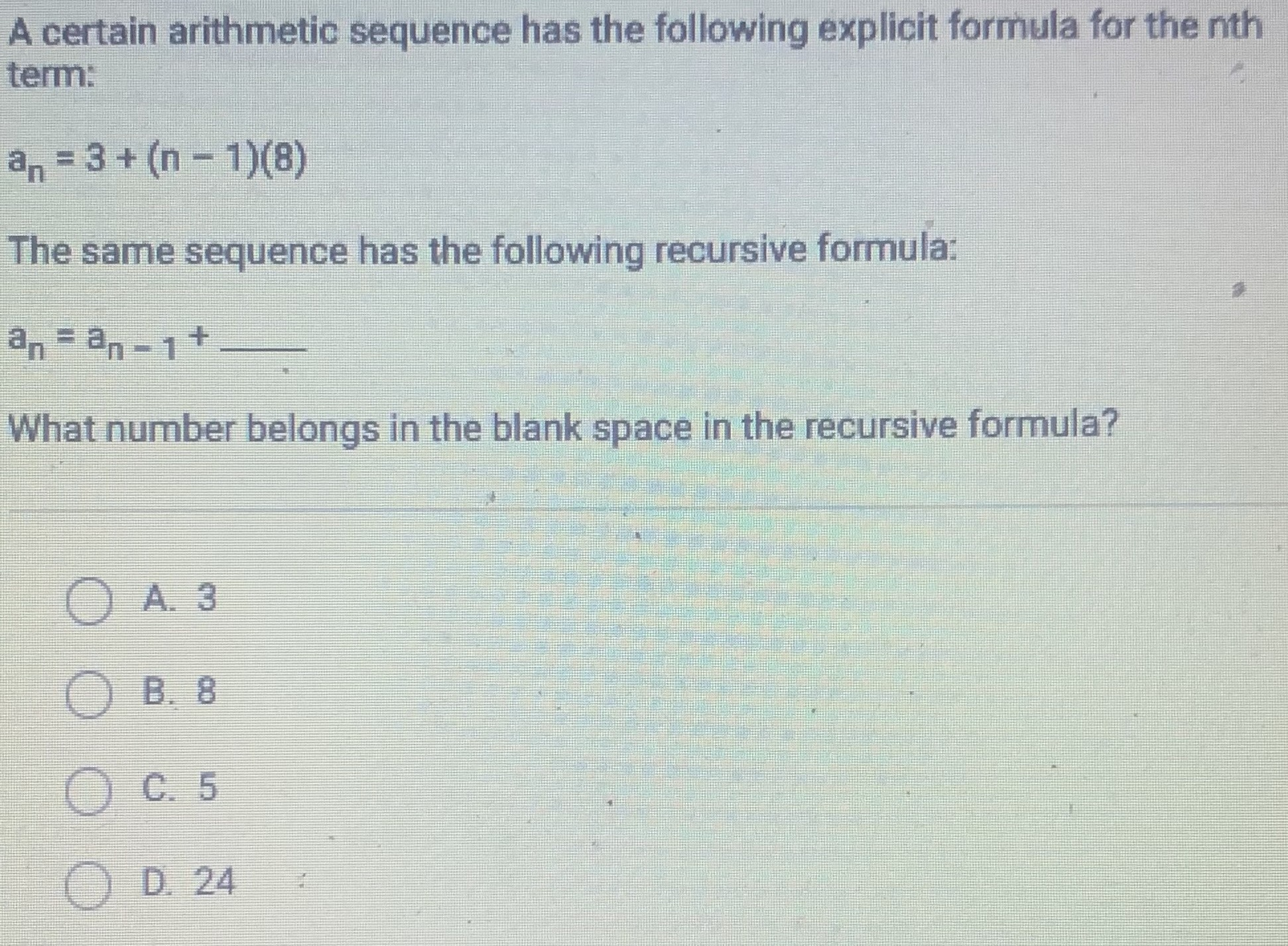### ¿Todavía tienes preguntas de matemáticas?

Pregunte a nuestros tutores expertos
Algebra
PreguntaA certain arithmetic sequence has the following explicit formula for the nth term:

$$a _ { n } = 3 + ( n - 1 ) ( 8 )$$

The same sequence has the following recursive formula:

$$a _ { n } = a _ { n - 1 } +$$

What number belongs in the blank space in the recursive formula?

(A. $$3$$ B B. $$8$$ C. $$5$$ D. $$24$$